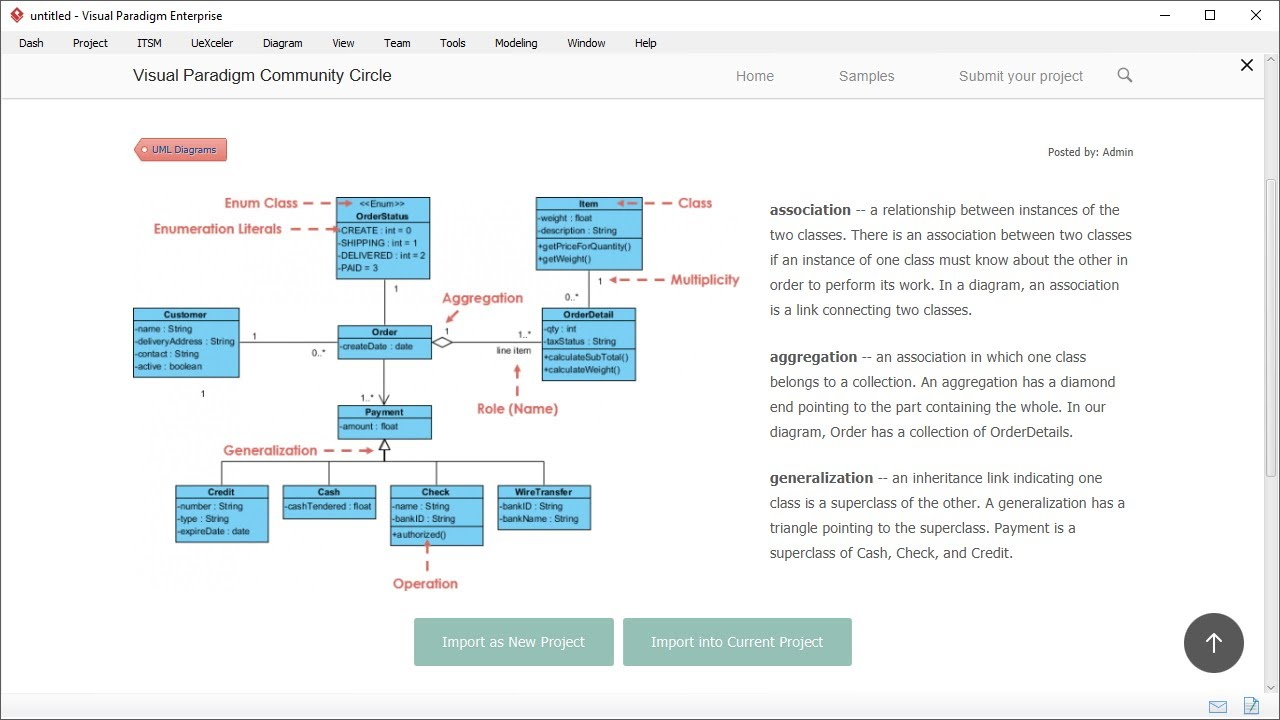# Free Diagram Templates and Examples

View and learn Visual Paradigm by importing selected diagrams straight into the Visual Paradigm platform for experimenting, practicing and making your own! You can also take advantage of the annotated examples in learning different modeling languages such as UML, ArchiMate, BPMN, etc.

Hi everyone, this is Jick from Visual Paradigm. In this demonstration I'm going to show you how you can learn a diagram from examples shared by others. To begin with, select Project, and Community Circle.

Visual Paradigm community circle contains many diagram templates and examples shared by our users. By scrolling down you see different categories like UML diagrams, BPMN diagrams, mind map, data flow diagrams, ArchiMate, PERT Chart, and etc.

If I click on a diagram category, the diagram examples of that type are listed, so I can click on an example.

There is a description that description this example, and the diagram.

The diagram is annotated. You can learn the diagram by studying the notations used.

Let's take a look at another example.

Class diagram. This is the sales order system example.

Besides learning you can also import an example into your project and modify it to make it to become yours. You can import into a new project or imported into your current project. Let's import into the current project.

The class diagram is imported into Visual Paradigm. I can make changes like to delete a class, rename class, add attributes and so forth.

This is the end of this video. Thank you for watching. Goodbye.

0 0 vote
Article Rating